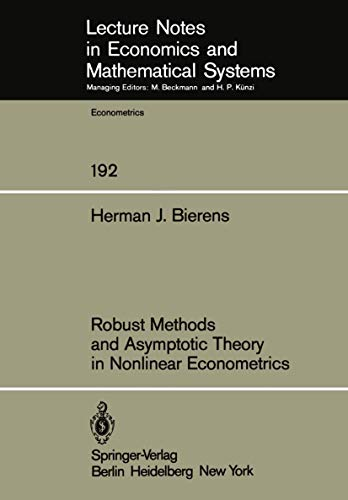Free Shipping in Australia
Proud to be B-Corp

# Robust Methods and Asymptotic Theory in Nonlinear Econometrics Herman J. Bierens\$198.79
Condition - New
Only 2 left

## Robust Methods and Asymptotic Theory in Nonlinear Econometrics Summary

### Robust Methods and Asymptotic Theory in Nonlinear Econometrics by Herman J. Bierens

This Lecture Note deals with asymptotic properties, i.e. weak and strong consistency and asymptotic normality, of parameter estimators of nonlinear regression models and nonlinear structural equations under various assumptions on the distribution of the data. The estimation methods involved are nonlinear least squares estimation (NLLSE), nonlinear robust M-estimation (NLRME) and non linear weighted robust M-estimation (NLWRME) for the regression case and nonlinear two-stage least squares estimation (NL2SLSE) and a new method called minimum information estimation (MIE) for the case of structural equations. The asymptotic properties of the NLLSE and the two robust M-estimation methods are derived from further elaborations of results of Jennrich. Special attention is payed to the comparison of the asymptotic efficiency of NLLSE and NLRME. It is shown that if the tails of the error distribution are fatter than those of the normal distribution NLRME is more efficient than NLLSE. The NLWRME method is appropriate if the distributions of both the errors and the regressors have fat tails. This study also improves and extends the NL2SLSE theory of Amemiya. The method involved is a variant of the instrumental variables method, requiring at least as many instrumental variables as parameters to be estimated. The new MIE method requires less instrumental variables. Asymptotic normality can be derived by employing only one instrumental variable and consistency can even be proved with out using any instrumental variables at all.

1 Introduction.- 1.1 Specification and misspecification of the econometric model.- 1.2 The purpose and scope of this study.- 2 Preliminary Mathematics.- 2.1 Random variables, independence, Borel measurable functions and mathematical expectation.- 2.1.1 Measure theoretical foundation of probability theory.- 2.1.2 Independence.- 2.1.3 Borel measurable functions.- 2.1.4 Mathematical expectation.- 2.2 Convergence of random variables and distributions.- 2.2.1 Weak and strong convergence of random variables.- 2.2.2 Convergence of mathematical expectations.- 2.2.3 Convergence of distributions.- 2.2.4 Convergence of distributions and mathematical expectations.- 2.3 Uniform convergence of random functions.- 2.3.1 Random functions. Uniform strong and weak convergence.- 2.3.2 Uniform strong and weak laws of large numbers.- 2.4 Characteristic functions, stable distributions and a central limit theorem.- 2.5 Unimodal distributions.- 3 Nonlinear Regression Models.- 3.1 Nonlinear least-squares estimation.- 3.1.1 Model and estimator.- 3.1.2 Strong consistency.- 3.1.3 Asymptotic normality.- 3.1.4 Weak consistency and asymptotic normality under weaker conditions.- 3.1.5 Asymptotic properties if the error distribution has infinite variance. Symmetric stable error distributions.- 3.2 A class of nonlinear robust M-estimators.- 3.2.1 Introduction.- 3.2.2 Strong consistency.- 3.2.3 Asymptotic normality.- 3.2.4 Properties of the function h(?). Asymptotic efficiency and robustness.- 3.2.5 A uniformly consistent estimator of the function h(?).- 3.2.6 A two-stage robust M-estimator.- 3.2.7 Some weaker results.- 3.3 Weighted nonlinear robust M-estimation.- 3.3.1 Introduction.- 3.3.2 Strong consistency and asymptotic normality.- 3.3.3 A two-stage weighted robust M-estimator.- 3.4 Miscellaneous notes on robust M-estimation.- 3.4.1 Uniform consistency.- 3.4.2 The symmetric unimodality assumption.- 3.4.3 The function ?.- 3.4.4 How to decide to apply robust M-estimation.- 4 Nonlinear Structural Equations.- 4.1 Nonlinear two-stage least squares.- 4.1.1 Introduction.- 4.1.2 Strong consistency.- 4.1.3 Asymptotic normality.- 4.1.4 Weak consistency.- 4.2 Minimum information estimators: introduction.- 4.2.1 Lack of instruments.- 4.2.2 Identification without using instrumental variables.- 4.2.3 Consistent estimation without using instrumental variables.- 4.2.4 Asymptotic normality.- 4.2.5 A problem concerning the nonsingularity assumption.- 4.3 Minimum information estimators: instrumental variable and scaling parameter.- 4.3.1 An instrumental variable.- 4.3.2 An example.- 4.3.3 A scaling parameter and its impact on the asymptotic properties.- 4.3.4 Estimation of the asymptotic variance matrix.- 4.3.5 A two-stage estimator.- 4.3.6 Weak consistency.- 4.4 Miscellaneous notes on minimum information estimation.- 4.4.1 Remarks on the function $$S_{ - n}^* (\theta |\gamma )$$.- 4.4.2 A consistent initial value.- 4.4.3 An upperbound of the variance matrix.- 4.4.4 A note on the symmetry assumption.- 5 Nonlinear Models with Lagged Dependent Variables.- 5.1 Stochastic stability.- 5.1.1 Stochastically stable linear autoregressive processes.- 5.1.2 Multivariate stochastically stable processes.- 5.1.3 Other examples of stochastically stable processes.- 5.2 Limit theorem for stochastically stable processes.- 5.2.1 A uniform weak law of large numbers.- 5.2.2 Martingales.- 5.2.3 Central limit theorem for stochastically stable martingale differences.- 5.3 Dynamic nonlinear regression models and implicit structural equations.- 5.3.1 Dynamic nonlinear regression models.- 5.3.2 Dynamic nonlinear implicit structural equations.- 5.4 Remarks on the stochastic stability concept.- 6 Some Applications.- 6.1 Applications of robust M-estimation.- 6.1.1 Municipal expenditure.- 6.1.2 An autoregressive model of money demand.- 6.2 An application of minimum information estimation.- References.

NLS9783540108382
9783540108382
3540108386
Robust Methods and Asymptotic Theory in Nonlinear Econometrics by Herman J. Bierens
New
Paperback
Springer-Verlag Berlin and Heidelberg GmbH & Co. KG
1981-06-01
198
N/A
Book picture is for illustrative purposes only, actual binding, cover or edition may vary.
This is a new book - be the first to read this copy. With untouched pages and a perfect binding, your brand new copy is ready to be opened for the first time